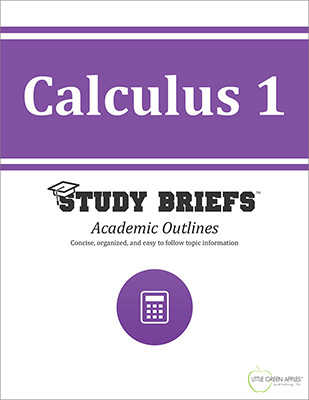# Calculus 1Look inside
• Format: eBook
• Edition: 2016
• Publisher: Little Green Apples Publishing LLC

Price: \$4.99

## Description:

The Calculus 1 Study Brief is intended as a quick reference for students of higher-level math and lifelong learners interested in advanced math topics. It offers a clear and concise conceptual framework (including: Key definitions) as it presents a varied selection of example equations in a visual format. The Study Brief provides example sets in the following categories: Functions (including: Polynomial, quadratic, rational, exponential, logarithmiic, and trigonometric); Limits (including: One-sided, Sandwich theorem, infinite limits, inequalities, and limits in trigonometry); Continuity (including: Intermediate and extreme value theorems); Derivatives (including: Trigonometry, inverse trigonometry, hyperbolic functions, exponential, and logarithmic functions); and Application of Derivatives (including: Rate of change, mean value theorem, and L'Hospital rule); and Integrals (indefinite and definite).

## Topics Covered:

• History of Calculus
• Functions
• Limits
• Continuity
• Derivatives
• Application of Derivatives
• Integrals
• Application of Integrals

Browse Subjects